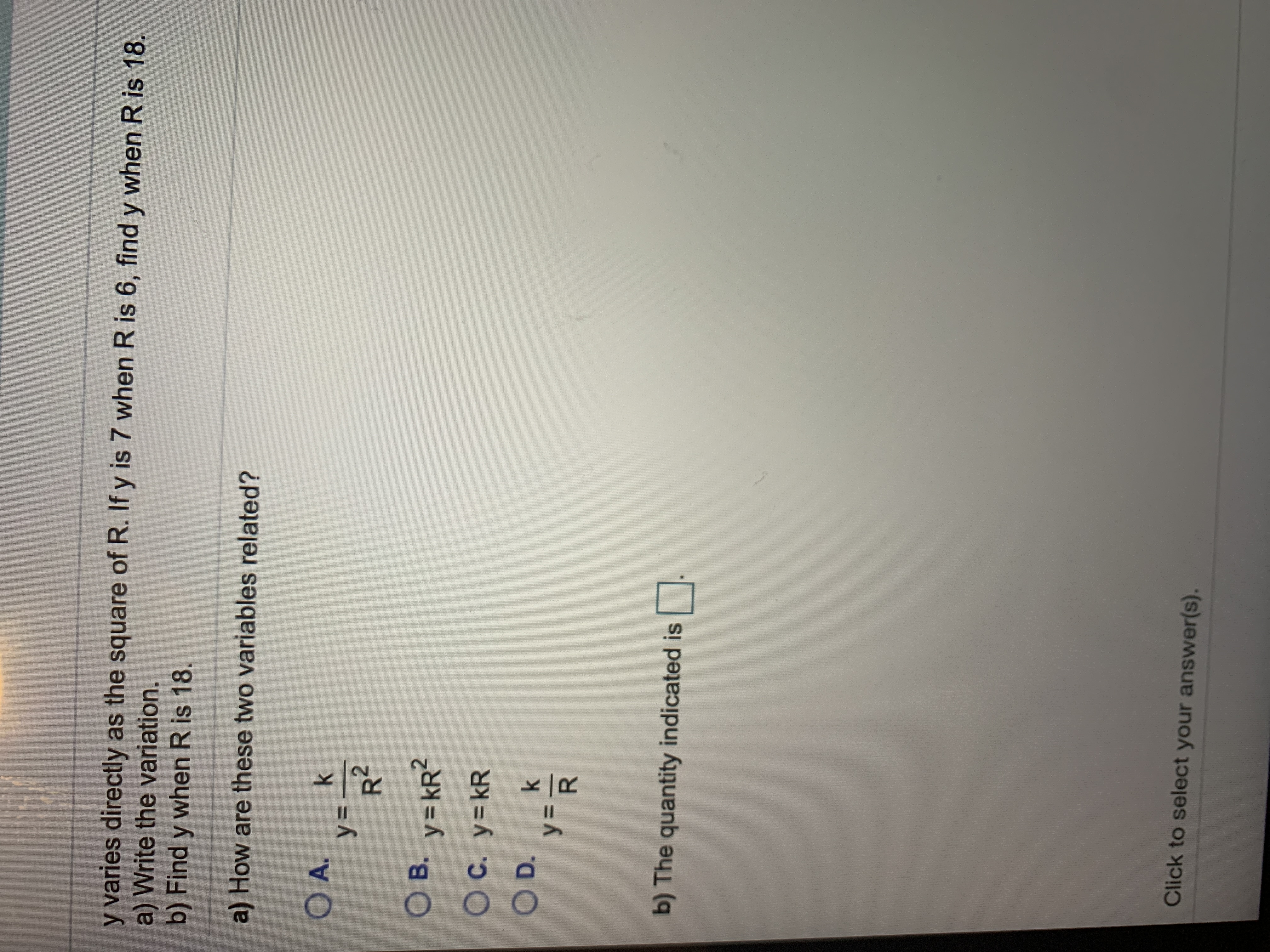# y varies directly as the square of R. If y is 7 when R is 6, find y when R is 18.a) Write the variation.b) Find y when R is 18.a) How are these two variables related?O A.kУ3-B. y kR2C. y kROD.kу-b) The quantity indicated isClick to select your answer(s).O O

Question
159 viewshelp_outlineImage Transcriptionclosey varies directly as the square of R. If y is 7 when R is 6, find y when R is 18. a) Write the variation. b) Find y when R is 18. a) How are these two variables related? O A. k У3- B. y kR2 C. y kR OD. k у- b) The quantity indicated is Click to select your answer(s). O O fullscreen
check_circle

Step 1

a) Given that y varies directly as the square of R

Then it can be written as y is proportional to R2

Step 2

Remove the proportionality sign

Step 3

b) It is given that y is 7 when R is 6

Plug ...

### Want to see the full answer?

See Solution

#### Want to see this answer and more?

Solutions are written by subject experts who are available 24/7. Questions are typically answered within 1 hour.*

See Solution
*Response times may vary by subject and question.
Tagged in

### Polynomials